• 向量叉积的模
千次阅读
2020-10-09 10:11:03

一：向量叉积

设两个点P（x1,y1,z1）,Q(x2,y2,z2)

叉积PxQ=(y1z2-y2z1，x2z1-x1z2，x1y2-x2y1)

代码实现（vb.net封成一个函数）:

Private Function Cross(ByVal Mat4() As Double, ByVal Mat5() As Double)
Dim Mat6() As Double
Mat6 = {Mat4(1) * Mat5(2) - Mat5(1) * Mat4(2), Mat4(2) * Mat5(0) - Mat4(0) * Mat5(2), Mat4(0) * Mat5(1) - Mat5(0) * Mat4(1)}
Return Mat6
End Function

二：向量叉积的模

向量积的模=两向量组成的平行四边形面积

|PxQ|= sqrt((x1y2-x2y1)^2+(y1z2-y2z1)^2+(z1x2-z2x1)^2)算法
更多相关内容
• 经典证明：向量叉积的几何意义 为什么以向量 (a, b) 和 (c, d) 为邻边，构成的平行四边形的面积正好是 ad – bc 呢？下图是一个非常漂亮的无字证明。 这是我在阅读 The Mathematical Mechanic: Using Physical ...

# 经典证明：向量叉积的几何意义

转自：http://www.matrix67.com/blog/archives/6217

为什么以向量 (a, b) 和 (c, d) 为邻边，构成的平行四边形的面积正好是 ad – bc 呢？下图是一个非常漂亮的无字证明。这是我在阅读 The Mathematical Mechanic: Using Physical Reasoning to Solve Problems 一书时受到启发并制作完成的。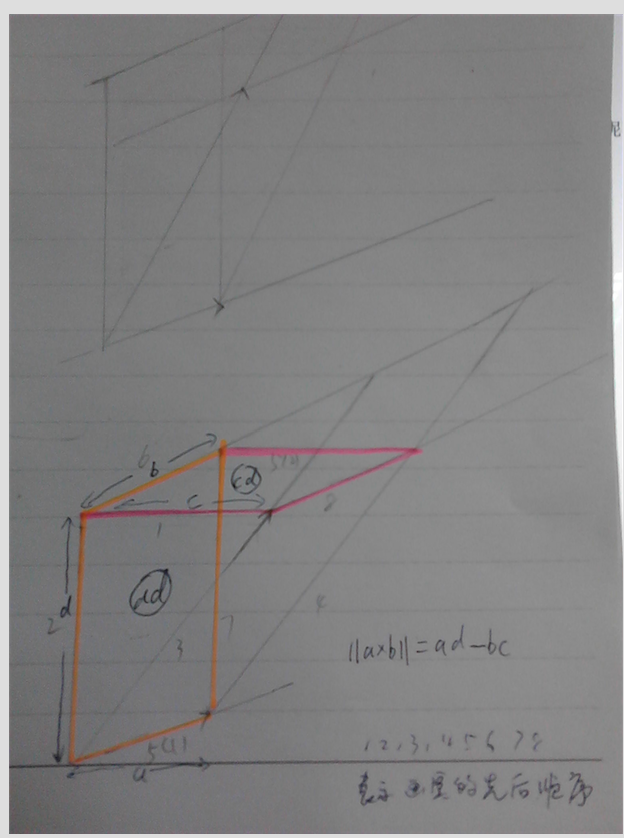展开全文• 向量叉积分配律简单证明 引入 叉积 向量叉积即向量积a×ba×ba×b，运算结果是一个向量，满足： 方向与aaa,bbb都垂直，符合右手法则； 等于∣a∣∣b∣sinθ|a||b|sinθ∣a∣∣b∣sinθ，几何意义为以aaa,bbb为...

# 向量叉积分配律简单证明

## 引入

##### 叉积
• 向量叉积即向量积 a × b a×b ，运算结果是一个向量，满足：
• 方向与 a a , b b 都垂直，符合右手法则；
• 模等于 ∣ a ∣ ∣ b ∣ s i n θ |a||b|sinθ ，几何意义为以 a a , b b 为邻边的平行四边形面积大小。
##### 坐标运算
• a = ( m , n ) a=(m,n) b = ( p , q ) b=(p,q)
• a × b a×b 的竖坐标 = m q − n p =mq-np （符号遵循右手法则）
##### 证明
• a = ( m , n ) a=(m,n) b = ( p , q ) b=(p,q)
• a = x 1 + y 1 = ( m , 0 ) + ( 0 , n ) a=x1+y1=(m,0)+(0,n) b = x 2 + y 2 = ( p , 0 ) + ( 0 , q ) b=x2+y2=(p,0)+(0,q)
• a × b a×b 的竖坐标 = ( x 1 + y 1 ) × ( x 2 + y 2 ) =(x1+y1)×(x2+y2)
= x 1 × x 2 + x 1 × y 2 + y 1 × x 2 + y 1 × y 2 =x1×x2+x1×y2+y1×x2+y1×y2
= x 1 × y 2 + y 1 × x 2 =x1×y2+y1×x2
= ( m , 0 ) × ( 0 , q ) + ( 0 , n ) × ( p , 0 ) =(m,0)×(0,q)+(0,n)×(p,0)
= m q − n p =mq-np
• 问题来了，这里的证明过程中，第一步直接把括号拆开，相当于默认叉积具有分配律，那么这一定是对的吗？
• 很多地方都没有给出证明，所以这里考虑对叉积分配律给出简单的证明。

## 证明

• 已知 a a , b b , c c 为平面内向量，求证： a × ( b + c ) = a × b + a × c a×(b+c)=a×b+a×c .
• 首先通过平移是向量起点重合，
• 从几何意义入手，需要满足以 a , b a,b 为邻边的平行四边形面积与以 a , c a,c 为邻边的平行四边形面积之和等于以 a , b + c a,b+c 为邻边的平行四边形面积（这里的面积可能为负值），• 显然，这么看难以证明。
• 那可以把 b b , c c 投影到与 a a 垂直的直线上，令投影后的向量分别为 b ′ b' , c ′ c' ，如图：• 由叉积的几何意义易得， a × b = a × b ′ a×b=a×b' a × c = a × c ′ a×c=a×c'
• a × b + a × c = a × b ′ + a × c ′ = − ∣ a ∣ ∣ b ′ ∣ + ∣ a ∣ ∣ c ′ ∣ = ∣ a ∣ ( ∣ c ′ ∣ − ∣ b ′ ∣ ) a×b+a×c=a×b'+a×c'=-|a||b'|+|a||c'|=|a|(|c'|-|b'|)• 同理又有 a × ( b + c ) = a × ( b + c ) ′ = ∣ a ∣ ∣ ( b + c ) ′ ∣ a×(b+c)=a×(b+c)'=|a||(b+c)'|
• 因为 ∣ c ′ ∣ − ∣ b ′ ∣ = ∣ ( b + c ) ′ ∣ |c'|-|b'|=|(b+c)'| ，所以 a × b ′ + a × c ′ = a × ( b + c ) ′ a×b'+a×c'=a×(b+c)' ，所以 a × ( b + c ) = a × b + a × c a×(b+c)=a×b+a×c
• 得证。
• 实际上，这样就相当于把原先的三个普通平行四边形转化为了三个矩形，便于证明。
展开全文向量
• 仅在三维空间，两个向量叉积才有定义，记作 u ^ v 定义为： u ^ v = ||u|| ||v|| sin(θ) n 其中，θ表示u 和 v 的夹角， ||u|| 和 ||v|| 分别是向量 u和v 的，n 则是u、v 所构成平面的法向（垂直于u、v平面的...

### 向量叉积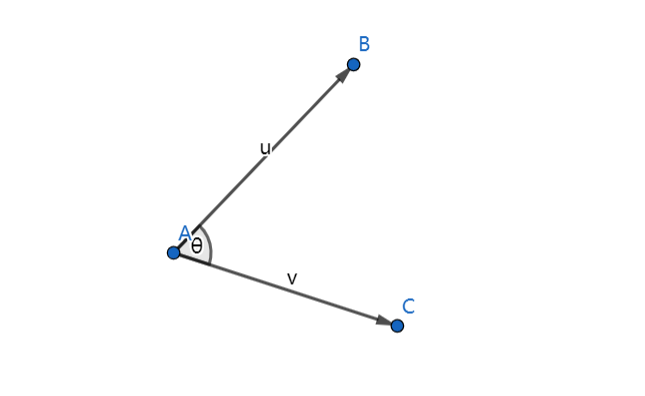仅在三维空间，两个向量的叉积才有定义，记作 u ^ v
定义为：
u ^ v = ||u|| ||v|| sin(θ) n
其中，θ表示uv 的夹角， ||u|| 和 ||v|| 分别是向量 uv 的模，n 则是uv 所构成平面的法向（垂直于uv平面的单位向量），方向由右手定则决定。

### 矩阵表示

叉积可以表示成如下行列式：其中， u = (u1, u2, u3)，v = (v1, v2, v3)，ijk为基向量，为三维坐标系的x, y, z方向的单位向量。
这个行列式可以使用拉普拉斯在展开和萨吕法则计算。
使用拉普拉斯展开可以沿第一展开为：使用萨吕法则可以展开为：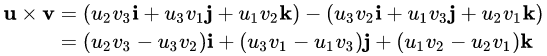### 几何意义

根据定义可以得出，向量叉积的几何意义是以uv为零边的平行四边形的有向面积。

### 应用

在计算机图形学中，向量叉乘的应用广泛。比如，判断线段的相对位置，线段相交，点在多边形内，求凸包等。

#### 判断两条线段的相对位置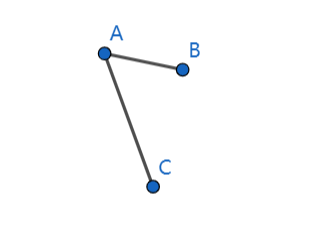在二维平面上有两条线段，分别是AB和AC，如何通过向量叉积来确定他们的相对位置关系
点A、B、C的坐标为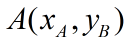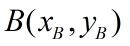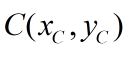首先构造两个向量AB和AC,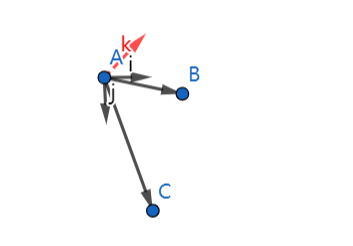由于二维空间不存在叉积的定义，所以引入z轴，将向量AB、AC扩展到三维空间，可以将二位向量可看作z轴恒为0的三维向量，
那么，两个向量叉积的则可表示为：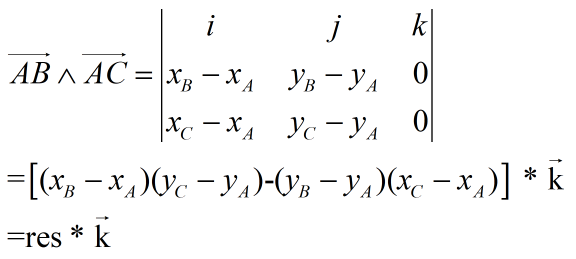res > 0, AC在AB的逆时针方向
res = 0, AB和AC共线
res < 0, AC在AB的顺时针方向

展开全文向量
• 文章目录一.预备知识 一.预备知识 ...①所得向量为： ∣a×b∣=∣a∣⋅∣b∣⋅sinθ|a×b|=|a|\cdot|b|\cdot sinθ∣a×b∣=∣a∣⋅∣b∣⋅sinθ 其中θθθ为向量a与向量b的夹角 ②所得向量的方向
• 后来打算写一篇叉积的证明时，却发现有些东西真的不好理解。设两个向量$\mathbf{a} = (x_1, y_1, z_1), \mathbf{b} = (x_2, y_2, z_2)$，两向量夹角为$\theta$，很多教材包括维基百科(Cross Product)等给出的定义都...叉积的证明
• 今天我们继续介绍向量的点积和叉积以及如何求向量的长度。下一篇再讲矩阵相关知识。两个向量A和B。则A和B的点积由如下公式给出：点积的几何意义是一个向量在另一个向量上的投影。AB可以理解A在B上的投影与B相乘，也...matlab 向量 matlab向量的模
• 叉乘（cross product）相对于点乘，叉乘可能更有用...上述结果是它的。在二维空间里，让我们暂时忽略它的方向，将结果看成一个向量，那么这个结果类似于上述的点积，我们有： A x B = |A||B|Sin(θ)然而角度 θ...
• 叉积 平面向量很好计算 例如： a=（x1,y1）,b=(x2,y2) 则 a×b=x1*y2-x2*y1; 空间向量 则用行列式则可计算 例如：a =(x1,y1,z1), b=(x2,y2,z2); | i j k | | x1 y1 z1 | | x2 y2 z
• 最近又回顾了一遍叉积，概念我们应该了解，几何上就是向量a和b，那么向量a和b的叉积得到向量c，向量c与向量a和b相互垂直，也就是说向量c垂直于向量a和b所在的平面，同时向量c的长等于向量a和b组成的平行四边形的...
• 详解互补滤波四元数中向量叉积与陀螺仪角速度补偿问题（Mahony算法） 置顶 2017年01月09日 22:12:45 阅读数：4317 标签： 互补滤波-四元数姿态解算四旋翼飞行器mahony更多 个人分类： 无人机 版权声明：本文...
• 向量的数量积（内积，点积）向量的向量积（外积，叉积），符合右手规则。，向量积是一个伪向量，定义其方向垂直于进行叉积的两个向量且满足...向量叉积的几何意义　计算矢量叉积是与直线和线段相关算法的核心部分...
•算法 机器学习 人工智能
• 向量加减法，向量的点积（乘），向量叉积（乘） 向量 是用来表示既有大小又有方向的量，不过向量在空间中没有具体的位置，通常用一个加粗的小写字母来表示一个向量，或者不加粗顶上带有小箭头的小写字母来表示 ...计算机图形学 向量 向量点积 向量叉积
• 很多做四轴的网友对互补滤波四元数姿态解算代码中的向量叉积和陀螺仪积分补偿问题有疑问，我也查了很多资料，写下这篇博文与大家共同学习。 先放一段互补滤波和四元数姿态解算的代码： /** * 6DOF 互补滤波姿...
• 定义：两个向量a和b的向量积（外积、叉积）是一个向量，记作a×b（这里“×”并不是乘号，只是一种表示方法，与“·”不同，也可记做“∧”）。若a、b不共线，则a×b的是：∣a×b∣=|a|·|b|·sin〈a，b〉；a×b的...
• ## 线性代数笔记4——向量3（叉积）

万次阅读 多人点赞 2018-01-05 13:52:16
向量叉积也叫外积、向量积、叉乘或矢量积。两个向量叉积是这样表示的：   　在二维空间内，向量A = 1, a2>，B = 1, b2>   　其几何意义就是以两个向量为边的平行四边形的面积，这在上篇文章中给出了...矢量积
• ## 向量积（叉积）

千次阅读 2017-08-17 17:53:08
a×b =absinθ*n ，ab为两向量长，θ是两向量的夹角，n是垂直二者的单位向量叉积的长度可以理解为以ab为邻边的平行四边形面积 叉积的运算 反交换律 a×b=-b×a 分配律 a×(b+c)=a×b+a×c 可与标量相乘...
• 长：（在这里θ表示两向量之间的夹角(共起点的前提下)（0° ≤ θ ≤ 180°），它位于这两个矢量所定义的平面上。） 方向：a向量与b向量向量积的方向与这两个向量所在平面垂直，且遵守右手定则。 ...
• 学到一半发现我不会判断向量的旋转方向，于是我又去学习了一下叉乘。作为蒟蒻的我看了半天看不懂，所以我决定写一个连我这样的蒟蒻都能看得懂的便于理解的指北（雾）。 先上结论：对于两根向量a(x1,y1),b(x2,y2)，...计算几何
• 向量复习（一）：定义、求解、四则运算、点积和叉积数学 向量
• 点乘比较简单，是相应元素的乘积的和： ...这里|A|我们称为向量A的(norm)。这样我们就和容易计算两条线的夹角： Cos(θ) = A·B /(|A|*|B|) 叉乘（cross product） 首先我们知道 ，对于向量u和v,...
• 1. 向量叉积与向量点积的区别 注：向量积 ≠向量的积（向量的积一般指点乘） 一定要清晰地区分开向量积（矢积）与数量积（标积）。见下表。 向量积（矢积）与数量积（标积）的区别 　 名称 标积 / 内积 / ...
• 向量叉积，可以用来判断几何中的点线位置关系，且频繁出现，非常重要 叉积 = a x b = x1y2-x2y1 二.如何使用 1.判断两个向量的位置关系 令p1=(x1,y1)，p2=(x2,y2) 若叉积大于0，则p1逆时针旋转到p2 若叉积等于0，...
• 在3D游戏开发中，经常用到向量的点积和叉积及其几何意义，为防止遗忘，在此记录一下。点积在数学中，点积(德语：Skalarprodukt、英语：Dot Product)又称数量积或标量积(德语：Skalarprodukt、英语：Scalar Product)......